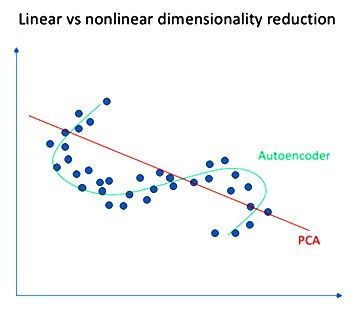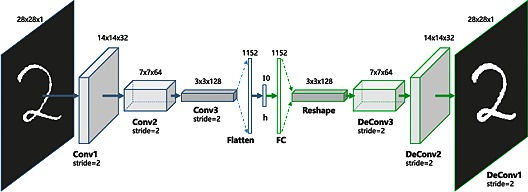top of page
Search

# DEEP AUTOENCODERS

Blog by Sandhya Reddy, MSRF intern

Artificial Intelligence encircles a wide range of technologies and techniques that enable computer systems to solve problems like Data Compression which is used in computer vision, computer networks, computer architecture, and many other fields. Autoencoders are unsupervised neural networks that use machine learning to do this compression for us.

## WHAT ARE AUTOENCODERS?

An autoencoder neural network is an Unsupervised Machine learning algorithm that applies backpropagation, setting the target values to be equal to the inputs. Autoencoders are used to reduce the size of our inputs into a smaller representation. If anyone needs the original data, they can reconstruct it from the compressed data. PCA does the same task.

AUTOENCODERS Vs PCA BECAUSE:Figure-1

• An autoencoder can learn non-linear transformations with a non-linear activation function and multiple layers.

• It doesn’t have to learn dense layers. It can use convolutional layers to learn which is better for video, image and series data.

• It is more efficient to learn several layers with an autoencoder rather than learn one huge transformation with PCA.

• An autoencoder provides a representation of each layer as the output.

• It can make use of pre-trained layers from another model to apply transfer learning to enhance the encoder/decoder.

Now let’s have a look at a few Industrial Applications of Autoencoders.

## APPLICATIONS OF AUTOENCODERS### Figure-2

• Image Colouring: Autoencoders are used for converting any black and white picture into a coloured image. Depending on what is in the picture, it is possible to tell what the colour should be.Figure-3

• Feature variation: It extracts only the required features of an image and generates the output by removing any noise or unnecessary interruption.Figure-4

• Dimensionality Reduction: The reconstructed image is the same as our input but with reduced dimensions. It helps in providing the similar image with a reduced pixel value.Figure-5

• Denoising Image: The input seen by the autoencoder is not the raw input but a stochastically corrupted version. A denoising autoencoder is thus trained to reconstruct the original input from the noisy version.Figure-6

• Watermark Removal: It is also used for removing watermarks from images or to remove any object while filming a video or a movie.

## ARCHITECTURE OF AUTOENCODERS

An Autoencoder consist of three layers:

1. Encoder

2. Code

3. DecoderFigure-7

• Encoder: This part of the network compresses the input into a latent space representation. The encoder layer encodes the input image as a compressed representation in a reduced dimension. The compressed image is the distorted version of the original image.

• Code: This part of the network represents the compressed input which is fed to the decoder.

• Decoder: This layer decodes the encoded image back to the original dimension. The decoded image is a lossy reconstruction of the original image and it is reconstructed from the latent space representation.Figure-8

The layer between the encoder and decoder, ie. the code is also known as Bottleneck. This is a well-designed approach to decide which aspects of observed data are relevant information and what aspects can be discarded. It does this by balancing two criteria :

• Compactness of representation, measured as the compressibility.

• It retains some behaviorally relevant variables from the input.

PROPERTIES OF AUTOENCODERS:

• Data-specific: Autoencoders are only able to compress data similar to what they have been trained on.

• Lossy: The decompressed outputs will be degraded compared to the original inputs.

• Learned automatically from examples: It is easy to train specialized instances of the algorithm that will perform well on a specific type of input.

HYPERPARAMETERS OF AUTOENCODERS:

There are 4 hyperparameters that we need to set before training an autoencoder:

• Code size: It represents the number of nodes in the middle layer. Smaller size results in more compression.

• Number of layers: The autoencoder can consist of as many layers as we want.

• Number of nodes per layer: The number of nodes per layer decreases with each subsequent layer of the encoder, and increases back in the decoder. The decoder is symmetric to the encoder in terms of the layer structure.

• Loss function: We either use mean squared error or binary cross-entropy. If the input values are in the range [0, 1] .then we typically use cross-entropy, otherwise, we use the mean squared error.

TYPES OF AUTOENCODERS

Convolution Autoencoders:

Autoencoders in their traditional formulation does not take into account the fact that a signal can be seen as a sum of other signals. Convolutional Autoencoders use the convolution operator to exploit this observation. They learn to encode the input in a set of simple signals and then try to reconstruct the input from them, modify the geometry or the reflectance of the image.Figure-9

Use cases of CAE:

• Image Reconstruction

• Image Colorization

• latent space clustering

• generating higher resolution images

Sparse Autoencoders:

Sparse autoencoders offer us an alternative method for introducing an information bottleneck without requiring a reduction in the number of nodes at our hidden layers. Instead, we’ll construct our loss function such that we penalize activations within a layer.Figure-10

Deep Autoencoders:

The extension of the simple Autoencoder is the Deep Autoencoder. The first layer of the Deep Autoencoder is used for first-order features in the raw input. The second layer is used for second-order features corresponding to patterns in the appearance of first-order features. Deeper layers of the Deep Autoencoder tend to learn even higher-order features.

A deep autoencoder is composed of two, symmetrical deep-belief networks-

1. First four or five shallow layers representing the encoding half of the net.

2. The second set of four or five layers that make up the decoding half.Figure-11

Use cases of Deep Autoencoders

• Image Search

• Data Compression

• Topic Modeling & Information Retrieval (IR)

Contractive Autoencoders:

A contractive autoencoder is an unsupervised deep learning technique that helps a neural network encode unlabeled training data. This is accomplished by constructing a loss term which penalizes large derivatives of our hidden layer activations with respect to the input training examples, essentially penalizing instances where a small change in the input leads to a large change in the encoding space.Figure-12

SIMPLE IMPLEMENTATION OF AUTOENCODERS USING TENSORFLOW IN PYTHON

1. Import the required libraries:

```import numpy as np
from keras.layers import Input, Dense
from keras.models import Model
from keras.datasets import mnist
import matplotlib.pyplot as plt```

2. Declaration of Hidden Layers and Variables:

This is the size of the encoded representations. 32 floats compression of factor 24.5, assuming the input is 784 floats

`encoding_dim = 32 `

This is our input placeholder

`input_img = Input(shape=(784,))`

"encoded" is the encoded representation of the input

`encoded = Dense(encoding_dim, activation='relu')(input_img)`

"decoded" is the lossy reconstruction of the input

`decoded = Dense(784, activation='sigmoid')(encoded)`

This model maps an input to its reconstruction

`autoencoder = Model(input_img, decoded)`

This model maps an input to its encoded representation

`encoder = Model(input_img, encoded)`

Create a placeholder for an encoded (32-dimensional) input

`encoded_input = Input(shape=(encoding_dim,))`

Retrieve the last layer of the autoencoder model

`decoder_layer = autoencoder.layers[-1]`

Create the decoder model

`decoder = Model(encoded_input, decoder_layer(encoded_input))`

Configure our model to use a per pixel binary crossentropy loss, and the Adadelta optimizer:

`autoencoder.compile(optimizer='adadelta', loss='binary_crossentropy')`

3. Preparing the input data (MNIST Dataset):

```(x_train, _), (x_test, _) = mnist.load_data()
# normalize all values between 0 and 1 and we will flatten the 28x28 images into vectors of size 784.
x_train = x_train.astype('float32') / 255.
x_test = x_test.astype('float32') / 255.
x_train = x_train.reshape((len(x_train), np.prod(x_train.shape[1:])))
x_test = x_test.reshape((len(x_test), np.prod(x_test.shape[1:])))
print x_train.shape
print x_test.shape```

4. Training Autoencoders for 50 epochs:

```autoencoder.fit(x_train, x_train,
epochs=50,
batch_size=256,
shuffle=True,
validation_data=(x_test, x_test))
# encode and decode some digits
# note that we take them from the *test* set
encoded_imgs = encoder.predict(x_test)
decoded_imgs = decoder.predict(encoded_imgs)```

5. Visualizing the reconstructed inputs and the encoded representations using Matplotlib

```n = 20 # how many digits we will display
plt.figure(figsize=(20, 4))
for i in range(n):
# display original
ax = plt.subplot(2, n, i + 1)
plt.imshow(x_test[i].reshape(28, 28))
plt.gray()
ax.get_xaxis().set_visible(False)
ax.get_yaxis().set_visible(False)
# display reconstruction
ax = plt.subplot(2, n, i + 1 + n)
plt.imshow(decoded_imgs[i].reshape(28, 28))
plt.gray()
ax.get_xaxis().set_visible(False)
ax.get_yaxis().set_visible(False)
plt.show()```For the complete code, checkout the following github link:

• https://github.com/sandhyareddy2001/DEEP-AUTOENCODERS

In Conclusion, in this blog the following topics were covered:

1. What are Autoencoders?

2. The need for Autoencoders

3. Applications of Autoencoders

4. Architecture of Autoencoders

5. Properties & Hyperparameters

6. Types of Autoencoders

7. Data Compression using Autoencoders (Demo)

## REFERENCES:

• https://www.edureka.co/blog/autoencoders-tutorial/

• https://blog.keras.io/building-autoencoders-in-keras.html

• https://www.jeremyjordan.me/autoencoders/

• https://wiki.pathmind.com/deep autoencoder#:~:text=A%20deep%20autoencoder%20is%20composed,make%20up%20the%20decoding%20half.

• https://towardsdatascience.com/deep-autoencoders-using-tensorflow-c68f075fd1a3